# Build a Text Table

In Tableau, you typically create text tables (also called cross-tabs or pivot tables) by placing one dimension on the Rows shelf and another dimension on the Columns shelf. You then complete the view by dragging one or more measures to Text on the Marks card.

A text table uses the text mark type. Tableau uses this mark type automatically if the view is constructed using only dimensions (assuming the mark type is set to Automatic). For more information about the text mark type, see Text mark.

To create a text table that shows sales totals by year and category, follow these steps:

1. Connect to the Sample - Superstore data source.
2. Drag the Order Date dimension to Columns.

Tableau aggregates the date by year and creates column headers.

3. Drag the Sub-Category dimension to Rows.

Now you can add a measure to the view to see actual data.

4. Drag the Sales measure to Text on the Marks card.

Tableau aggregates the measure as a sum.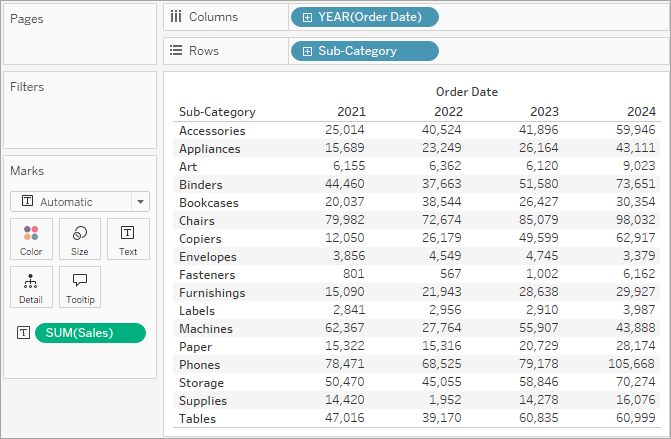Tableau uses text as the mark type. Each cell in the table displays the sum of sales for a particular year and sub-category.

We can see that the chairs and phones sub-categories had the highest sales in every year.

5. Drag the Region dimension to Rows and drop it to the left of Sub-Category. A small triangle will appear to indicate that the new field will be inserted to the left of the existing field.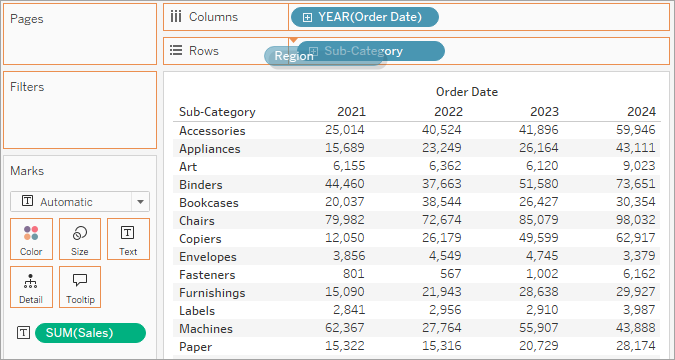The view now breaks down sales by region, in addition to year and sub-category.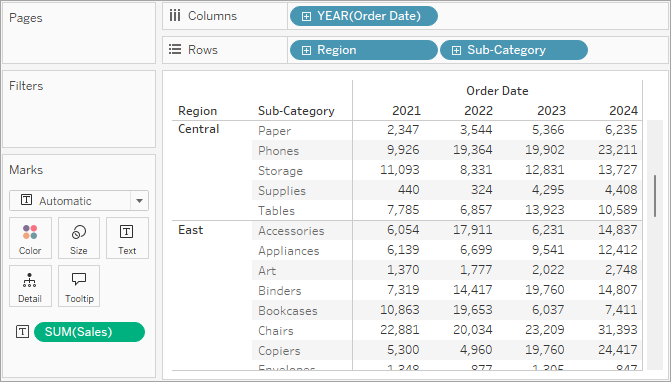Regions are listed alphabetically. You can drag Region to the right of Sub-Category to organise the view first by sub-category, and then by region.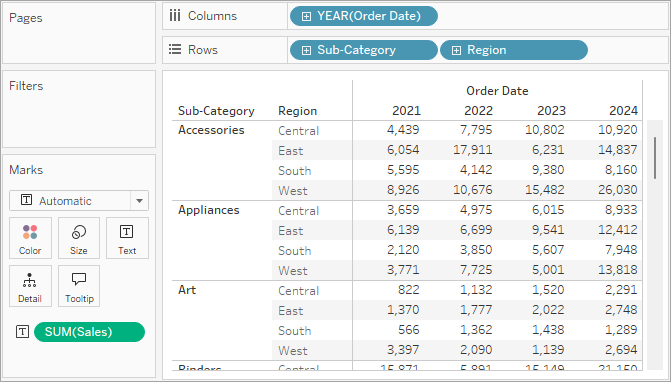You can use a table calculation to show percentages of the total instead of raw dollar values. First, you must determine how to frame the calculation.

In this case, there are three dimensions in the view: Order Date, Sub-Category, and Region.

You could show percentages of total for a single dimension, but that can be unwieldy. For example, if you show percentages just by region, the percentages would be calculated across the two remaining dimensions: Sub-Category (there are 17 sub-categories) and Year(Order Date) (there are 4 years). So you would be dividing the total 17 x 4 = 68 ways. That would make for some tiny percentages.

Instead, show percentages using two dimensions: Year(Order Date) and Region. Then the percentages are calculated on the remaining dimension, Sub-Category, that is, you calculate percent of total within each highlighted area shown below.The dimensions that you use to frame your calculation are called the addressing fields, and the fields in which you run your calculation are the partition fields.

6. To create a table calculation to show percentages, right-click (control-click on Mac) the SUM(Sales) field on the Marks card, and then select Add Table Calculation.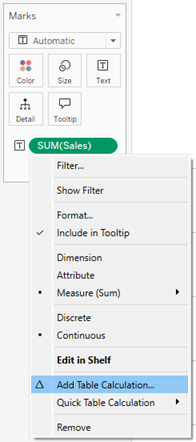7. In the Table Calculation dialog box, set Calculation Type to Percent of Total.

The options in the dialog box change depending on the type of calculation you choose.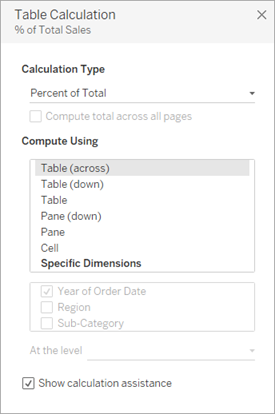8. For the Calculation definition, select Pane (Down), and then close the Table Calculation dialog box.

Now we see percentages calculated within each sub-category, duplicated for each year within each region. The numbers within each highlighted area add up to 100%.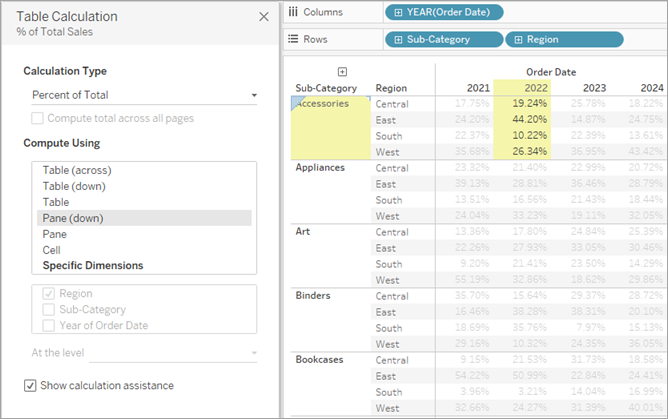Pane (Down) is the appropriate choice because it specifies that the calculation should be performed from top to bottom within each pane of the table. The table has two vertical dimensions, so Table (Down) would have calculated the percent of total from top to bottom for the entire table, ignoring the Region dimension.

The pane is always the finest level of detail for the relevant direction (across or down). If you had three dimensions on the vertical axis, you might have had to use field names to define the calculation, because only the dimension furthest to the left on the Rows shelf (defined as Table) and the dimension furthest to the right (defined as Pane) could be captured with the structural options.

### Check your work! Watch steps 1-8 below:Thanks for your feedback! There was an error submitting your feedback. Try again or send us a message.